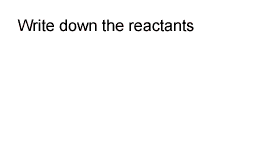Naming precipitates A precipitate is an insoluble solid product formed from the mixing of two solutions. Consider the reaction shown on the right. A white insoluble solid (precipitate) is formed when lead nitrate is mixed with sodium carbonate.Now let's say we mixed two clear solutions of silver nitrate and sodium carbonate. How do we predict the name of the precipitate? Follow the steps listed below and shown in the animation on the right. Step 1 Write the names of each solution Step 2 Swap the first names Step 3 Discard the name that contains any of the following - sodium - nitrate - ammonium - potassium. Step 4 The remaining name is usually the precipitate.Knowing the name of the precipitate we can easily write the formula. Click for a refresher on how to write ionic formulae. Give the name and formula of the precipitate formed when the following solutions are mixed Refer to the table of electrovalencies. 1) Lead nitrate ( Pb(NO3)2 and potassium dichromate ( K2Cr2O4) Solution2) Barium chloride (BaCl2) and potassium dichromate ( K2Cr2O4) Solution 3) Silver nitrate ( Ag(NO3) and potassium dichromate ( K2Cr2O4) Solution 4) Lead nitrate ( Pb(NO3)2 and copper sulfate (CuSO4) Solution 5) Lead nitrate ( Pb(NO3)2 and sodium carbonate (Na2CO3) Solution 6) Copper sulfate (CuSO4) + potassium iodide (KI) Solution 7) Copper sulfate (CuSO4) + potassium dichromate ( K2Cr2O4) Solution Try some more exercises